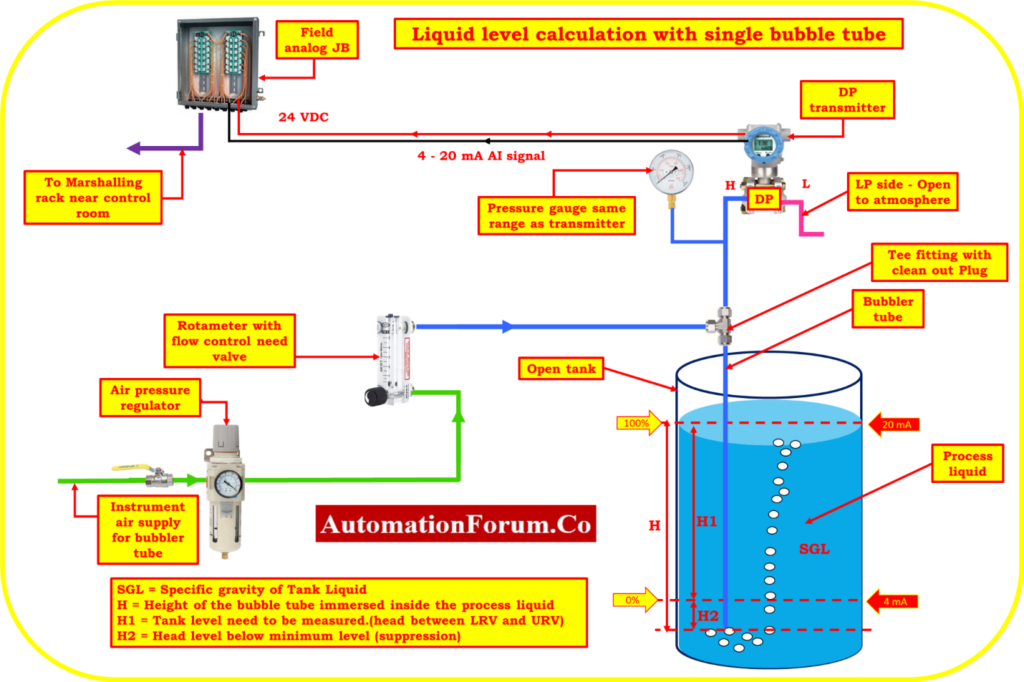# Bubbler method level calculation – Liquid level calculation (Transmitter Installed with single Bubble tube)Examples of standard calculations for measuring liquid levels in an open tank with a single Bubble tube Transmitter Installation are provided in this section.

It is important to keep in mind that the upper-range values (URV) and lower-range values (LRV) relate to the highest and minimum measurements that are intended, respectively. The Calibrated Span is equal to the URV minus the LRV. The output at LRV greater than 0% represents the value of the suppression.

## Abbreviations

The following acronyms are utilized in this directive.

LRV = Lower-range value (a measurement that’s output is 4 mA).

URV = Upper-range value (a measurement with a 20 mA output).

SG = A liquid’s specific gravity (relative density). Water has a specific gravity of 1.00 in both traditional and SI systems.

HW= Equivalent Headlevel pressure of Water

## Formulas for Converting Specific Gravity for All Liquids

Specific gravity = Density [lb/ft3 ] / 62.4

Specific gravity = Density [kg/m3] / 1.00

## Liquid Level Calculations (Transmitter installed with single Bubble Tube)

SGL = Specific gravity of Tank Liquid

H = Height of the bubble tube immersed inside the process liquid

H1 = Tank level need to be measured.(head between LRV and URV)

H2 = Head level below minimum level (suppression)

Calibrated Range of the transmitter = LRV to URV

where: SGL = Specific gravity of tank process liquid

HW= Equivalent Headlevel pressure of Water

Span value of the transmitter  = H1*SGL

Equivalent Head level pressure of Water(HW) at Lower range value

HW at LRV = H1*SGL = Suppression

Equivalent Head level pressure of Water(HW) at Higher range value

Hw at URV = (H1 +H2)*SGL

## Example calculation- Transmitter installed with single Bubble Tube for level measurement

The transmitter installed in open tank with following parameters

Height level measured between LRV and URV is

H1= 2000mm,

Head level distance below minimum level(LRV) (suppression)

H2 = 200mm,

The process liquid’s specific gravity is

SGL = 0.8

Find the calibration range of the transmitter?

## Span value calculation

Span value = H1*SGL

Span = (2000)*(0.8)

Span value of the transmitter = 1600 mmH2O

## LRV calculation (4mA)

HW at LRV = H1*SGL

Hw at LRV = (200)*(0.8)

Hw at LRV= 160 mmH2O = Suppression

## URV calculation (20mA)

Hw at URV = (H1 +H2)*SGL

Hw at URV = (2000 + 200) (0.8)

Hw at URV = 1760 mmH2O

Calibration Range of the transmitter = 160 to 1760 mmH2O